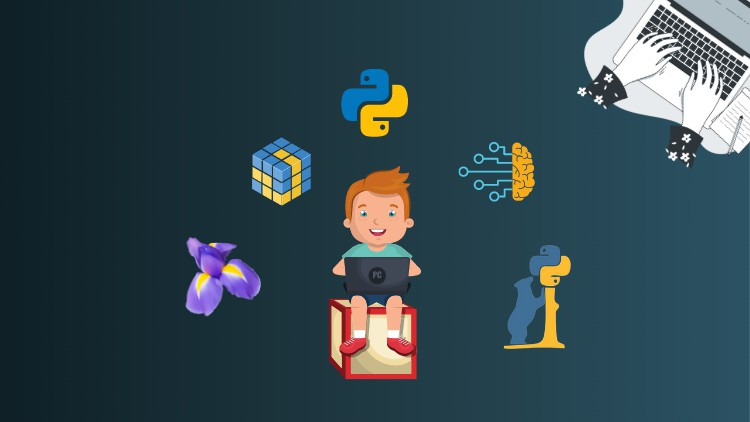# Python for Machine Learning with Numpy, Pandas & Matplotlib## Learn to Code in Python and How to use NumPy, Pandas, Matplotlib and Seaborn by real time Machine Learning project.

What you will learn

Learn Fundamentals of Python for effectively using Data Science

Use Python for Data Science and Machine Learning

Refresh Python basics with crash course

Make use of Numpy and Pandas to implement numerical algorithms

Data Manipulation

Learn to use NumPy for Numerical Data

Array and Matrix manipulation Library NumPy

Learn to use Numpy for Data Manipulation

Numpy functions

Understand and code using the Numpy stack

Learn to use Pandas for Data Analysis

Three important data structure of pandas : Series, Data Frame, Panel

Data Visualization

Visualise Data using Matplotlib and Seaborn

Description

Are you ready to start your path to becoming a Data Scientist or ML Engineer?

This comprehensive course will be your guide to learning how to use the power of Python to analyze data, create beautiful visualizations, and use powerful machine learning algorithms!

Data Scientist has been ranked the number one job on Glassdoor and the average salary of a data scientist is over \$120,000 in the United States according to Indeed! Data Science is a rewarding career that allows you to solve some of the world’s most interesting problems!

This course is designed for both beginners with some programming experience or experienced developers looking to make the jump to Data Science!

This comprehensive course is comparable to other Data Science bootcamps that usually cost thousands of dollars, but now you can learn all that information at a fraction of the cost! With over 100 HD video lectures and detailed code notebooks for every lecture this is one of the most comprehensive course for data science and machine learning on Udemy!

We’ll teach you how to program with Python, how to create amazing data visualizations, and how to use Machine Learning with Python! Here a just a few of the topics we will be learning:

1. Programming with Python
2. NumPy with Python
3. Using pandas Data Frames to solve complex tasks
4. Use pandas to handle Excel Files
5. Visualize Data using Matplotlib and Seaborn

## English

Language

Content

Python Crash Course

Python Crash Course

Numpy & Pandas for Data Science

Numpy & Pandas : Part 1

Numpy & Pandas : Part 2

Data Visualization

Data Visualizaton : Part 1

Data Visualizaton : Part 2